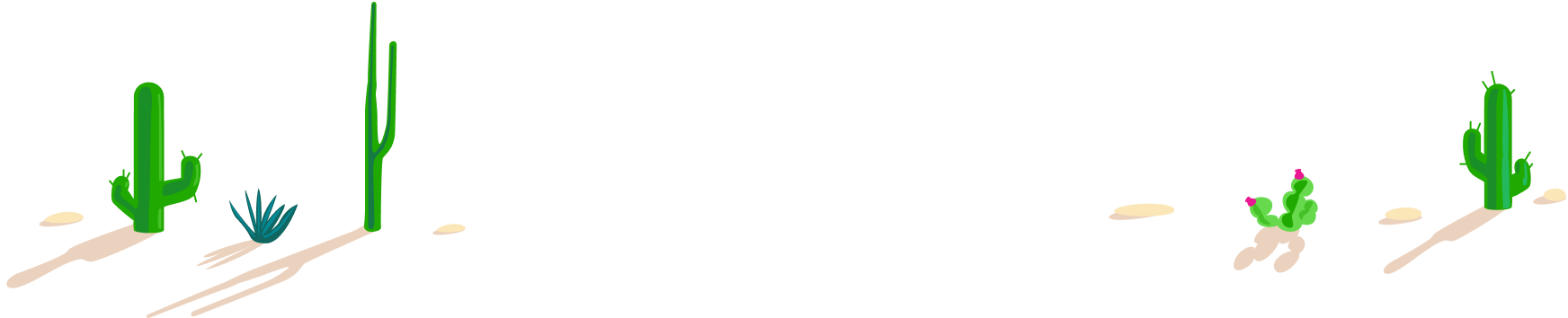# 100 Day Summer Challenge

## 100 problems in 100 days. #100problems

Day 13# 13 of 100: Operator Search

Algebra Level 1

$\large 10\; \Box\; 10\; \Box\; 10\; \Box\; 10=101$

Is it possible to fill each square in with an arithmetic operation $(+, -, \times, \div)$ so that this becomes a true equation?You'd be surprised how many people forget about the order of operations...

Bonus question: Replace 101 with another number between 0 and 200 that makes this problem impossible.
Difficult follow-up bonus: How many different numbers is it possible to make with four 10's and three arithmetic operations?